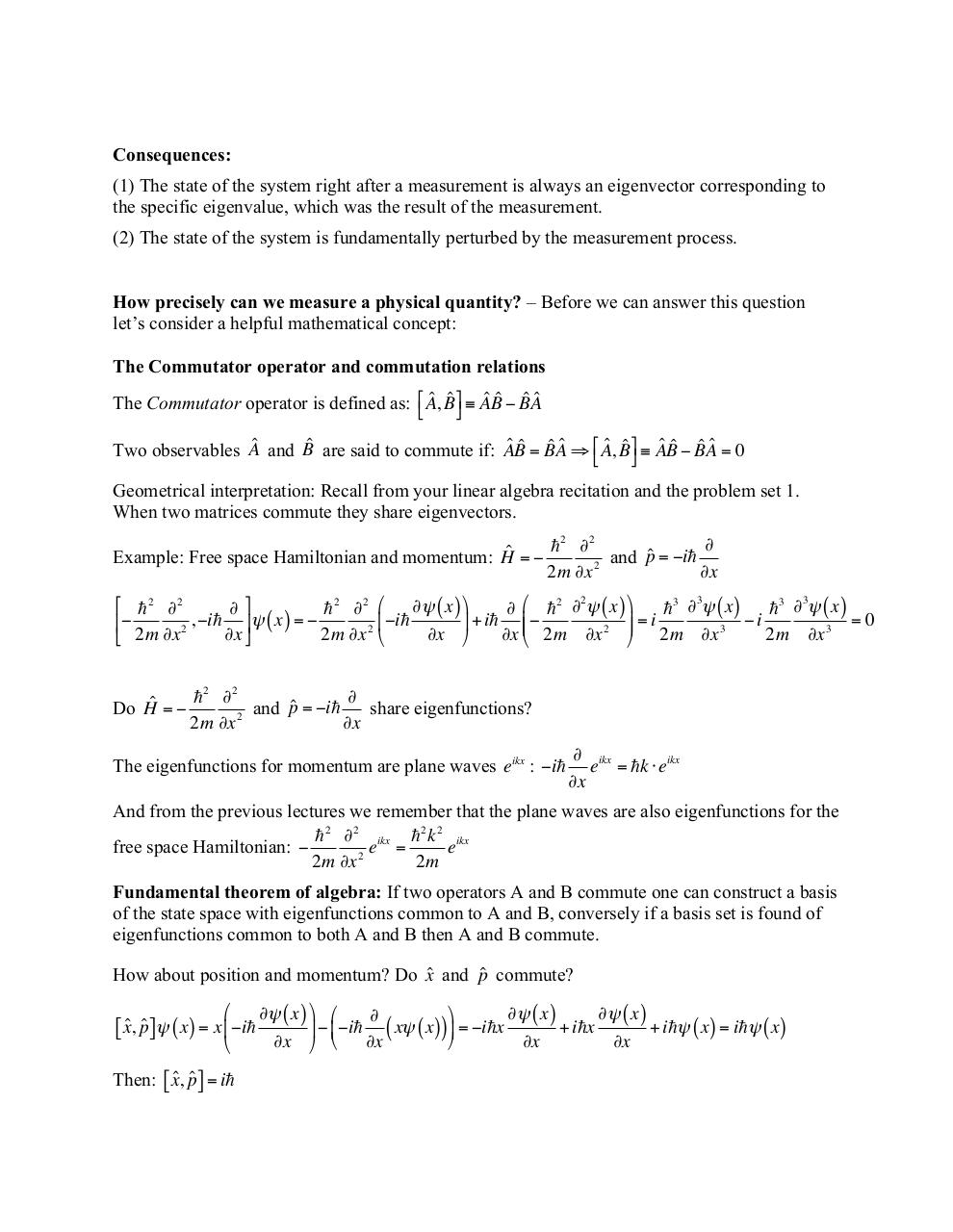L7+QM+Measurements+and+Constants+of+Motion.pdfPage 1 2 3 4 5 6 7 8 9

Text preview

Consequences:
(1) The state of the system right after a measurement is always an eigenvector corresponding to
the specific eigenvalue, which was the result of the measurement.
(2) The state of the system is fundamentally perturbed by the measurement process.
How precisely can we measure a physical quantity? – Before we can answer this question
let’s consider a helpful mathematical concept:
The Commutator operator and commutation relations

ˆ Bˆ # ≡ Aˆ Bˆ − Bˆ Aˆ
The Commutator operator is defined as: !&quot; A,
\$
ˆ Bˆ \$ ≡ Aˆ Bˆ − Bˆ Aˆ = 0
Two observables Aˆ and Bˆ are said to commute if: Aˆ Bˆ = Bˆ Aˆ ⇒ &quot;# A,
%
Geometrical interpretation: Recall from your linear algebra recitation and the problem set 1.
When two matrices commute they share eigenvectors.

 2 ∂2
Example: Free space Hamiltonian and momentum: Hˆ = −
and pˆ = −i
2
∂x
2m ∂x
2
3
3
#  2 ∂2
∂&amp;
 2 ∂2 ) ∂ψ ( x ) ,
∂ ) 2 ∂ ψ ( x ) ,
3 ∂ ψ ( x )
3 ∂ ψ ( x )

,
−i
ψ
x
=

−i
+
i

=
i

i
=0
+
.
+
.
(
)
%
(
2
∂x '
2m ∂x 2 *
∂x ∂x +* 2m ∂x 2 .- 2m ∂x 3
2m ∂x 3
\$ 2m ∂x

 2 ∂2
Do Hˆ = −
and pˆ = −i
share eigenfunctions?
2
∂x
2m ∂x
The eigenfunctions for momentum are plane waves eikx : −i

∂ ikx
e = k ⋅ eikx
∂x

And from the previous lectures we remember that the plane waves are also eigenfunctions for the
 2 ∂2 ikx  2 k 2 ikx
free space Hamiltonian: −
e =
e
2m ∂x 2
2m
Fundamental theorem of algebra: If two operators A and B commute one can construct a basis
of the state space with eigenfunctions common to A and B, conversely if a basis set is found of
eigenfunctions common to both A and B then A and B commute.
How about position and momentum? Do xˆ and pˆ commute?
#

[ x,ˆ pˆ ] ψ ( x ) = x % −i
\$

ˆ pˆ ] = i
Then: [ x,

∂ψ ( x ) &amp; #
∂ψ ( x )
∂ψ ( x )
&amp;

+ ix
+ iψ ( x ) = iψ ( x )
( − % −i ( xψ ( x )) ( = −ix
'
∂x ' \$
∂x
∂x
∂x